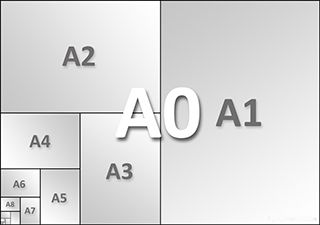# Practice on Toph

Participate in exhilarating programming contests, solve unique algorithm and data structure challenges and be a part of an awesome community.

# Story of A4

By mridul_sust · Limits 1s, 512 MB

You all know the A4 papers. But do you know why are they called A4 or why are they so special? Well, there's a very interesting fact about these. If you cut an A4 paper in half (the larger side should be cut), you'll get two mini versions of A4 papers. That means the ratio of height to width of the small papers will be the same as the original A4 paper.

Since this is called A4, maybe you can guess this is a part of a series of A papers. Yes, you are right ! The paper with 1 square meter of area is called A0. If you cut this into half, you'll get two A1 papers. And from one A1 you'll get two A2, from one A2, you'll get two A3, from one A3, you'll get two A4 and so on... Please refer to the figure to clearly understand how you should make the cuts. So, now you know the story behind the so familiar name A4.We want to make a name to size calculator. And you can now forget the traditional size of an A0 paper (1 square meter area). This may vary now.

You are given the width ($W_m$ ) of an $A_m$ paper in centimeters. You need to find the sum of the dimensions (in centimeters) of an $A_n$ paper.

Consider the smaller side as width and larger side as height.

## Input

The input starts with an integer T (T ≤ 100 ) denoting the number of test cases.
Each of the next T lines contains three integers m, $W_m$ and n (0 ≤ n ≤ m ≤ 70, 1 ≤ $W_m$ ≤ 1000 ).

## Output

For each case, output a single line containing a floating point number denoting the sum of the width and the height of an $A_n$ paper.
Print exactly 4 digits after decimal point.

Please refer to the sample input/output section for better understanding.

## Sample

InputOutput
2
3 15 0
5 35 3

102.4264
168.9949


### Statistics

28% Solution Ratio

user.725518Earliest, Aug '20

user.725518Fastest, 0.0s

user.725518Lightest, 131 kB

steinumShortest, 238B

### Submit